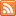# Content Tagged “Numerical Measures”Numerical summaries obtained from data which describe particular aspects of the distribution of the variable.## Case C-C

Published: Aug 21st, 2012

Two Categorical Variables Contingency Tables Finding Conditional (Row and Column) Percents Let’s Summarize CO-4: Distinguish among different measurement scales, choose the appropriate descriptive and inferential statistical methods based on these distinctions, […]## Case C-Q

Published: Aug 21st, 2012

Categorical Explanatory and Quantitative Response Let’s Summarize CO-4: Distinguish among different measurement scales, choose the appropriate descriptive and inferential statistical methods based on these distinctions, and interpret the results. LO 4.20: Classify […]Published: Aug 21st, 2012

Introduction Range Inter-Quartile Range (IQR) Standard Deviation Properties of the Standard Deviation Choosing Numerical Measures Let’s Summarize CO-4: Distinguish among different measurement scales, choose the appropriate descriptive and inferential statistical methods […]## Measures of Center

Published: Aug 21st, 2012

Introduction Mean Median Comparing the Mean and the Median Let’s Summarize CO-4: Distinguish among different measurement scales, choose the appropriate descriptive and inferential statistical methods based on these distinctions, and interpret […]

## Interactive Applet – One Quantitative Variable

Published: Aug 19th, 2012

This interactive online data analysis applet is part of the online content for Introduction to the Practice of Statistics, 8th Edition (Moore and McCabe). Choose one of the datasets in the list and click […]

## One Quantitative Variable

Published: Aug 19th, 2012

Distribution of One Quantitative Variable Graphs Numerical Measures CO-4: Distinguish among different measurement scales, choose the appropriate descriptive and inferential statistical methods based on these distinctions, and interpret the results. Video: One […]## One Categorical Variable

Published: Aug 4th, 2012

Distribution of One Categorical Variable Numerical Measures Visual or Graphical Displays Pie Chart Bar Chart Pictograms Let’s Summarize CO-4: Distinguish among different measurement scales, choose the appropriate descriptive and inferential statistical […]## Unit 1: Exploratory Data Analysis

Published: Jul 30th, 2012

The Big Picture Exploratory Data Analysis Important Features of Exploratory Data Analysis Examining Distributions CO-1: Describe the roles biostatistics serves in the discipline of public health. CO-6: Apply basic concepts of […]